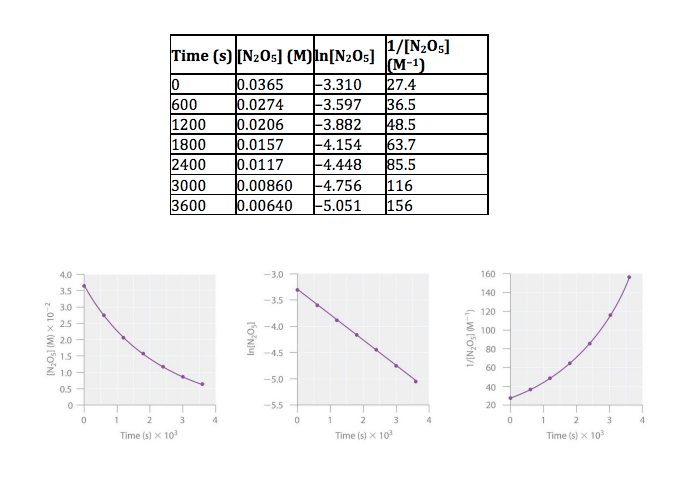# Problem: Dinitrogen pentoxide (N2O5) decomposes to NO2 and O2 at relatively low temperatures in the following reaction: 2N2O5(aq) → 4NO2(aq) + O2(g) This reaction is carried out in a CCl 4 solution at 45°C. The data are described in the table as well as the plots of the concentration versus t, ln concentration versus t, and 1/concentration versus t and results are below. Determine the rate law and calculate the rate constant. a. Zero order/ 5.3 x10-5 s -1     b. Second order/ 4.8 x 10−4 s −1 M-1      c. First order/5.3 x 10-5 s -1 M-1 d. Second order/ 2.9 x 10−4 s −1 M-1               e. First order/4.8 x 10−4 s −1

###### FREE Expert Solution
93% (481 ratings)
###### Problem Details

Dinitrogen pentoxide (N2O5) decomposes to NO2 and O2 at relatively low temperatures in the following reaction:

2N2O5(aq) → 4NO2(aq) + O2(g)

This reaction is carried out in a CCl 4 solution at 45°C. The data are described in the table as well as the plots of the concentration versus t, ln concentration versus t, and 1/concentration versus t and results are below. Determine the rate law and calculate the rate constant.

a. Zero order/ 5.3 x10-5 s -1     b. Second order/ 4.8 x 10−4 s −1 M-1      c. First order/5.3 x 10-5 s -1 M-1

d. Second order/ 2.9 x 10−4 s −1 M-1               e. First order/4.8 x 10−4 s −1What scientific concept do you need to know in order to solve this problem?

Our tutors have indicated that to solve this problem you will need to apply the Identifying Reaction Order concept. If you need more Identifying Reaction Order practice, you can also practice Identifying Reaction Order practice problems.

What is the difficulty of this problem?

Our tutors rated the difficulty ofDinitrogen pentoxide (N2O5) decomposes to NO2 and O2 at rela...as medium difficulty.

How long does this problem take to solve?

Our expert Chemistry tutor, Jules took undefined undefined to solve this problem. You can follow their steps in the video explanation above.

What professor is this problem relevant for?

Based on our data, we think this problem is relevant for Professor Hogan's class at LSU.